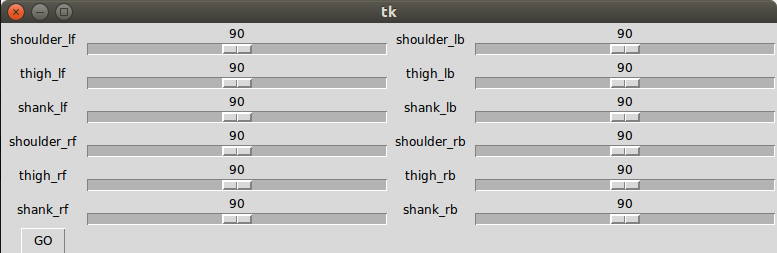I wrote an article on ROS servo motor control two years ago, and the method was successfully applied in my previous 9g Quadruped Robot project. Later, I figured that if I don’t need servo motor pose feedback, or handling servo motor group movement in the microcontroller level, things can be much eaiser by simply using an Adafruit PCA9685, Arduino and Python.

The hardware setup is pretty simple:

• The PCA9685 board is connected with Arduinot Uno using I2C, they share ground, and PCA9685 in my setting is also using the 5V from Arduino (which can be from anywhere else).
• My Arduino is connected with my desktop PC using USB cable.
• My servo motors (RDS3135) need high voltage (~8V) and high current (~3A peak), thus they are not plugged onto the PCA9685 directly, instead they are using external power supply, the signal pins are connected to PCA9685 of course, and they need to share ground.I used Python to make a simple GUI that can test control all the servo angles. I will use it to test the joint servo motor angles later thus the servos are named as shoulder, thigh, shank and etc.The Arduino firmware:

``````#include <Wire.h>

const int SERVOMIN = 150;
const int SERVOMAX = 600;
const int SERVONUM = 12;

static char serial_in;

static int servo_angles[SERVONUM];

void setUpServos()
{
for (int i = 0; i < SERVONUM; i ++)
{
servo_angles[i] = 90;
}
}

void setServoAngle(int servo_ind, float angle)
{
int pwm_value = angle / 180 * (SERVOMAX - SERVOMIN) + SERVOMIN;
pwm.setPWM(servo_ind, 0, pwm_value);
}

void setAllServosAngle()
{
for (int i = 0; i < SERVONUM; i ++)
{
setServoAngle(i, servo_angles[i]);
}
}

void checkSerial()
{
if (Serial.available() > 13)
{
if (serial_in == '#')
{
for (int i = 0; i < SERVONUM; i ++)
{
servo_angles[i] = Serial.parseInt();
}
if (serial_in == 'e')
{
setAllServosAngle();
}
}
}
}

void setup()
{
Serial.begin(9600);

pwm.begin();
pwm.setPWMFreq(60);  // Analog servos run at ~60 Hz updates

setUpServos();

delay(10);
}

void loop()
{
checkSerial();
}
``````

The Python script:

``````#!/usr/bin/python2

import Tkinter as tk
import serial

class actionGUI:
def __init__(self, master):
self.master = master

try:
self.robot_ser = serial.Serial('/dev/ttyACM0', 9600)
except:
print "Cannot connect to the robot"

self.servo_names = ['shoulder_lf', 'thigh_lf', 'shank_lf', 'shoulder_rf', 'thigh_rf', 'shank_rf',
'shoulder_lb', 'thigh_lb', 'shank_lb', 'shoulder_rb', 'thigh_rb', 'shank_rb']
self.servo_pose = [0, 0, 0, 0, 0, 0, 0, 0, 0, 0, 0, 0]

self.bias_list = [0, 0, 0, 0, 0, 0, 0, 0, 0, 0, 0, 0]
#self.dir_list = [-1,1,-1, -1,1,1, -1,-1,1, -1,1,-1]
self.dir_list = [1,1,1, 1,1,1, 1,1,1, 1,1,1]

self.servo_scales = []
for r, servo_name in enumerate(self.servo_names):
tk.Label(text = servo_name, width = 10).grid(row = r%6, column = 0 if r<6 else 2)
servo_scale = tk.Scale(self.servo_pose[r], orient = tk.HORIZONTAL, width = 10, to = 180, length=300)
servo_scale.set(90)
servo_scale.grid(row = r%6, column = 1 if r<6 else 3)
self.servo_scales.append(servo_scale)

r = r%6 + 1
self.go_button = tk.Button(master, text = "GO", command = self.go_callback)
self.go_button.grid(row = r, column = 0)

def go_callback(self):
#send the action goal
command = '#'
for i, servo_name in enumerate(self.servo_names):
pose = (self.servo_scales[i].get()-90)*self.dir_list[i] + 90 + self.bias_list[i]
command = command + ',' + str(pose)
command = command + 'e'
self.robot_ser.write(command)
try:
self.robot_ser.inWaiting()
except:
print "Lost connection!"
print 'Command sent!'
print command

def main():
root = tk.Tk()
app = actionGUI(root)
root.mainloop()

if __name__ == "__main__":
main()
``````